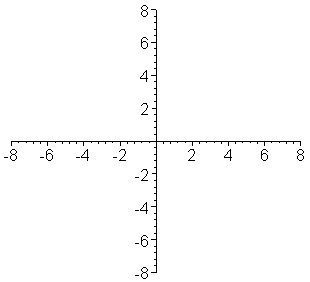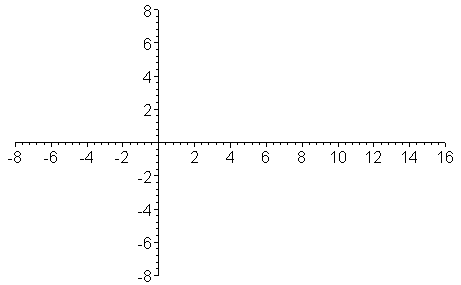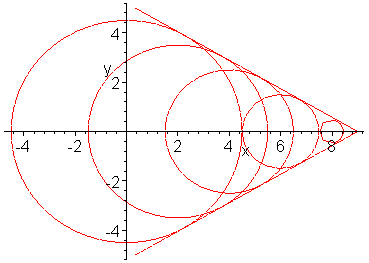### Doppler Effects

 Doppler EffectsApparent change in the wave frequency (or in the period of periodic events) due to motion of wave source and/or observer is known as Doppler effect. In the 17th century (well before Doppler effect was predicted), Roemer (Danish astronomer) estimated the speed of light from the change in the revolution period of one of Jupiter's moons. When the earth is approaching Jupiter, the period is shortened, while receding, the period is elongated. The first animation shows wave patterns (surfaces of constant phase) emitted by a moving wave source. Wavelengths in front of the moving source are squeezed (shortened) according to and those behind are elongated,where cw is the wave (sound) velocity and Vs is the velocity of the wave source. Therefore, the frequency of a wave source approaching (receding from) an observer is higher (lower) than the "true" frequency,Similar effect occurs when the observer is moving. For sound waves, the velocities of source and observer are well defined relative to air, the wave medium. For stationary sound source and moving observer, the sound speed appears to change (the wavelength remains the same). Therefore, the Doppler shifted frequency for a stationary sound source heard by a moving observer is given by where Vo is the velocity of the observer relative to air, + sign for the case of approaching and ?sign for the case of receding.For electromagnetic waves in vacuum, the velocity with respect to the wave medium (vacuum) is ill defined. In Doppler effect of electromagnetic waves,only the relative velocity between wave source and observer matters, where is the relative velocity between the wave source and observer normalized by the velocity of light c. The factor in the numerator, is due to intrinsic Doppler shift caused by time dilation effect.Some objects (e.g., supersonic planes) can move faster than sound waves. Animation below shows wave fronts emitted by a supersonic object when the Mach number is 2. No waves can exist outside the cone created by the object. When the cone surface hits an observer, sudden arrival of a sharp pulse is detected as shock wave. In vacuum, nothing can travel faster than light. However, in material media (glass, plastic, etc.), electromagnetic waves propagate in general at a speed slower than c. Therefore, if a relativistic charged particle with a velocity close to c enters, say, a plastic block, electromagnetic shock wave is emitted. This phenomenon is called Cerenkov radiation and is in wide use for detecting energetic particles.Doppler effect. Volocity of wave source = one half of the wave velocity. Wavelengths in front of the source are squeezed while those behind are elongated. animate({[u*sin(t),u*cos(t),t=-Pi..Pi], [(u-1)*sin(t)*Heaviside(u-1)+.5,(u-1)*cos(t)*Heaviside(u-1),t=-Pi..Pi],  [(u-2)*sin(t)*Heaviside(u-2)+1.,(u-2)*cos(t)*Heaviside(u-2),t=-Pi..Pi],  [(u-3)*sin(t)*Heaviside(u-3)+1.5,(u-3)*cos(t)*Heaviside(u-3),t=-Pi..Pi],  [(u-4)*sin(t)*Heaviside(u-4)+2.,(u-4)*cos(t)*Heaviside(u-4),t=-Pi..Pi],  [(u-5)*sin(t)*Heaviside(u-5)+2.5,(u-5)*cos(t)*Heaviside(u-5),t=-Pi..Pi],  [(u-6)*sin(t)*Heaviside(u-6)+3.,(u-6)*cos(t)*Heaviside(u-6),t=-Pi..Pi],  [(u-7)*sin(t)*Heaviside(u-7)+3.5,(u-7)*cos(t)*Heaviside(u-7),t=-Pi..Pi]},  u=0..8,view=[-8..8,-8..8],frames=40,color=red);Velocity of wave source = twice of the wave velocity. Formation of shock wave. animate({[u*sin(t),u*cos(t),t=-Pi..Pi], [(u-1)*sin(t)*Heaviside(u-1)+2,(u-1)*cos(t)*Heaviside(u-1),t=-Pi..Pi],  [(u-2)*sin(t)*Heaviside(u-2)+4,(u-2)*cos(t)*Heaviside(u-2),t=-Pi..Pi],  [(u-3)*sin(t)*Heaviside(u-3)+6,(u-3)*cos(t)*Heaviside(u-3),t=-Pi..Pi],  [(u-4)*sin(t)*Heaviside(u-4)+8,(u-4)*cos(t)*Heaviside(u-4),t=-Pi..Pi],  [(u-5)*sin(t)*Heaviside(u-5)+10,(u-5)*cos(t)*Heaviside(u-5),t=-Pi..Pi],  [(u-6)*sin(t)*Heaviside(u-6)+12,(u-6)*cos(t)*Heaviside(u-6),t=-Pi..Pi],  [(u-7)*sin(t)*Heaviside(u-7)+14,(u-7)*cos(t)*Heaviside(u-7),t=-Pi..Pi]},  u=0..8,view=[-8..16,-8..8],frames=40,color=red);> interface(plotdevice=inline):  with(plots):  implicitplot({x^2+y^2=4.5^2,(x-2)^2+y^2=3.5^2,(x-4)^2+y^2=2.5^2,  (x-6)^2+y^2=1.5^2,(x-8)^2+y^2=0.5^2,x+1.732*y=9,x-1.732*y=9},x=-5..9,y=-5..5,numpoints=1000);Ċ
Jason Lin,
2018年9月6日 凌晨12:43